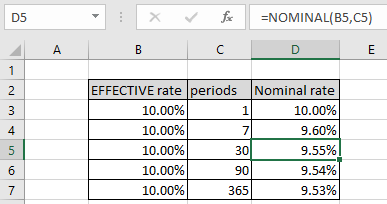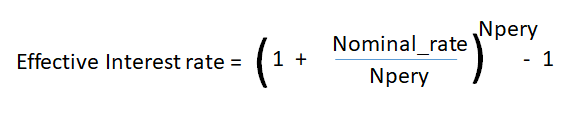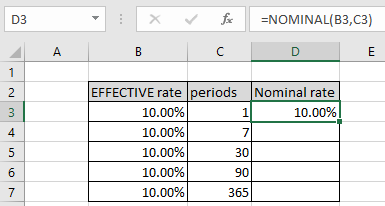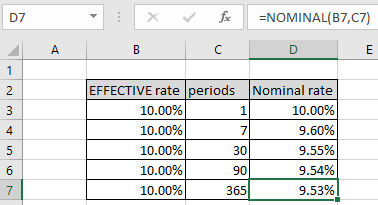# How to use the NOMINAL function in ExcelIn this article, we will learn How to use the NOMINAL function in Excel.

Scenario :

When working with financial statements, sometimes we need to use the nominal interest rate instead of effective interest rate. Nominal interest rate refers to the interest rate before taking inflation into account. Nominal can also refer to the advertised or stated interest rate on a loan, without taking into account any fees or compounding of interest.

There are two forms of Interest rate used in terms of loan.

1. Nominal Interest rate : The nominal interest rate doesn’t take into account the annual compounding period.
2. Effective Interest rate : The effective Interest rate does take into account the annual compounding period and thus returns the accurate measures of Interest charges.

The numerical calculation relation between the two is shown below.Here Npery is number of compounding periods per year

The above formula can be used either way to calculate effective interest rate given nominal interest rate and npery value.

NOMINAL function in Excel

NOMINAL function returns the interest rate for the given effective rate argument and compounding period per year (npery) argument. Let's understand the formula with the arguments used.

NOMINAL function syntax :

 =NOMINAL (effect_rate, npery)

effective rate : effective rate given as percentage or in decimals.

npery : number of compounding period per year

Example :

All of these might be confusing to understand. Let's understand this function using an example. Here we are given a 10% effective rate with different compounding periods per year value to show the change in nominal rate w.r.t to npery value.

Use the formula:

 =NOMINAL(B3,C3)As you can see if the npery is equal to 1. Copy the formula to the rest of cells using the shortcut Ctrl + D or dragging down from the formula from the right bottom of the cell.As you can see the change in nominal rate due to change in compounding periods. Generally, effective interest rate is used as it takes into account the annual compounding period and thus returns the accurate measures of Interest charges.

Here are all the observational notes regarding using the formula.
Notes :

1. Npery argument is truncated to nearest integers by the formula itself.
2. If npery equal to 1 then nominal rate = effective rate
3. The function generates #VALUE! Error if arguments to the function is non - numeric.
4. The function generates #NUM! Error if:
• The value of effective_rate is less than or equal to 0.
• The value of npery is less than one.

Hope you understood how to use the NOMINAL function in Excel. Find more articles on financial formulas and related functions here. If you liked our blogs, share it with your friends on Facebook. And also you can follow us on Twitter and Facebook. We would love to hear from you, do let us know how we can improve, complement or innovate our work and make it better for you. Write to us at info@exceltip.com.

Related Articles

How to use the EFFECT function in excel : returns the effective annual interest rate, given a nominal interest rate and the number of compounding periods per year. Effective annual interest rate is the interest rate actually earned due to compounding.

How to use the MIRR function in excel : returns the Modified interest rate of return for the financial data having Investment, finance rate & reinvestment_rate using the MIRR function in Excel.

How to use the XIRR function in excel : returns the Interest rate of return for irregular interval using the XIRR function in Excel

Excel PV vs FV function : find Present Value using PV function and future value using FV function in Excel.

How to use the RECEIVED function in excel : calculates the amount which is received at maturity for a bond with an initial investment (security) and a discount rate, there are no periodic interest payments using the RECEIVED function in excel.

How to use the NPER function in excel : NPER function to calculate periods on payments in Excel.

How to use the PRICE function in excel : returns the price per \$100 face value of a security that pays periodic interest using the PRICE function in Excel.

Popular Articles:

How to use the IF Function in Excel : The IF statement in Excel checks the condition and returns a specific value if the condition is TRUE or returns another specific value if FALSE.

How to use the VLOOKUP Function in Exceln : This is one of the most used and popular functions of excel that is used to lookup value from different ranges and sheets.

How to Use SUMIF Function in Excel : This is another dashboard essential function. This helps you sum up values on specific conditions.

How to use the COUNTIF Function in Excel : Count values with conditions using this amazing function. You don't need to filter your data to count specific values. Countif function is essential to prepare your dashboard.

Terms and Conditions of use

The applications/code on this site are distributed as is and without warranties or liability. In no event shall the owner of the copyrights, or the authors of the applications/code be liable for any loss of profit, any problems or any damage resulting from the use or evaluation of the applications/code.